## Monday, April 27, 2009

### The Dot and Cross Products

The dot product and cross product of two vectors are tools which are heavily used in physics. As such, they are typically introduced at the beginning of first semester physics courses, just after vector addition, subtraction, etc. Although they are not strictly required for these intro courses (see , for example), they make the development and computations of work and energy, torque, and electromagnetism far simpler.

Unfortunately, they are consistently introduced in an awful way: by straight definition. That is, using the dot product for example, for two vectors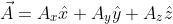and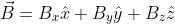they say something like
We define the dot product between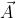andas: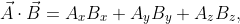or,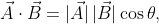where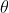is the angle between them.
Then, for the cross product, either they use an equation like the latter of the above two equations coupled with the ``right-hand rule,'' or a strange algebraic combination of the components ofand, often ``simplified'' with help of a startling determinant.1 See , , ,  and  as a few examples. Although a few of these give a geometric interpretation after the fact, it is usually in passing, and does not really contribute to their discussion. These approaches are not limited to textbooks, either. See  for an in-class lecture example.

In these examples, the dot product is introduced first and then the cross product. From one standpoint this makes some sense -- the dot product is definitionally simpler and usually easier to calculate. However, from a conceptual standpoint, I think this order is backwards. Furthermore, in my experience, students, by and large, miss the physical and graphical significance of these definitions, and upon encountering the concepts of work or torque later on, take the resulting expressions purely as definitions as well.2 This is yet another example of the fact that definition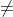explanation.

Personally, it is my inclination to wait to introduce these products until they're needed, thus motivating the discussion in the first place. However, I do understand the notion of ``getting it over with,'' and, it's possible that introducing them as abstract concepts lends to easier application of the concepts to general problems. In any case, my discussion follows the latter approach (for better insertion into standard texts) and presupposes understanding of vector basics: addition, decomposition, etc..

A better way
The Cross Product
(a) Geometrical view of the cross product as the parallelogram area.

(b) Graphical derivation of area for two 2D arbitrary vectors, from .
Figure 1: The 2D cross product of vectorsand.

Say we have two vectorsandwith lengths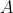and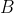, and we want to find something which is a measure of how much ofis perpendicular to. Looking at Fig. 1(a), we can see that the area of the parallelogram sided by the two vectors is such a measure. The area of a parallelogram is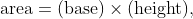(1)
which, for our case, is the same as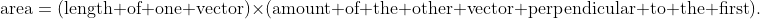(2)
That is, you can only have an area if you have a ``base'' and a ``height'' perpendicular to the base. Thus area is a good measure of perpendicularity.3

There are two different ways of calculating this area. If the angle between the two vectors is, as in Fig. 1(a), we see that, choosingas the ``base'' we can write the ``height'' as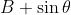. Alternatively, choosingas the base, we write the perpendicular part as. Then the area is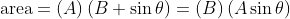.
(3)
However, if we don't know angle between them, we're not completely out of luck. If you look at Fig. 1(b), you can see that for a simple, two-dimensional case, we can express the area in terms of the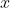and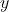components ofand: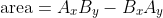(4a)
Of course, I could just have easily labeled the axesand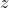which would give a different area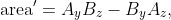(4b)
orand, which would give yet another area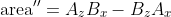(4c)

If all we've done is relabel our axes, keepingandfixed, then we wouldn't expect the size of these areas to be different -- and they're not. However, although the amount of area is the same, in a way the areas are different in that they're facing different directions in each case. So, we need a way to distinguish these three areas from each other, and from an arbitrarily oriented area. What we'll do is pick a vector perpendicular to bothto-- and thus perpendicular to the area of the parallelogram -- with magnitude equal to the area. We'll call this vector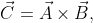(5)
and say it's the result of a ``cross product'' ofand. However, in principle, we have a choice of two such perpendicular vectors. In Fig. 1, for example, we could choose the vector pointing in either the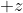or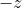direction. Additionally, this arbitrariness can be seen in choosing whether to measure the angle in (3) fromtoor vise-versa.

So, as a matter of convention, we'll decide to always measure angles from the first term in the cross product (in (5)) such that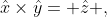(6)
so if the fingers in your right hand point along the little arcs we draw for angles, your thumb points in the direction that this vector goes. Thus,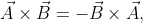(7)
since your hand would curl in the other direction. This is called the ``Right-Hand Rule.'' Then, the areas we discussed in equations (4) become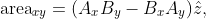(8a)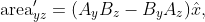(8b)
and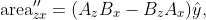(8c)
where the subscripts tell us which coordinate plane the two crossed vectors are in. Thus, the cross product represents how much these two vectors point in perpendicular directions, and is a signed area vector perpendicular to the plane described byand.

(a) Geometrical view of the 3D cross product as the parallelogram area.
(b) Looking at the area from the xy-plane (dashed outline), the yz-plane (shaded) and the zx-plane (solid).
Figure 2: The 3D cross product of vectorsandand the decomposed area.

So far, though, we've only discussed vectors which have only two coplanar components. But it's fairly straightforward to generalize to arbitrary 3D vectors. See Fig. 2(a), for example. Here the area vector, and hence the cross product vector, is pointing in a complicated direction. However, we know we can decompose any vector into its,andcomponents, and this area vector is no different: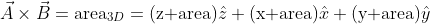(9)

All we need to do is find out how much area is pointing in each direction. To do that, look at Fig. 2(b). This picture shows what the area between the two vectors looks like if we look only at two coplanar components at a time -- in other words the,andcomponents of the area. But we already know what each of these areas are from (8)! So, then we can combine these equations and write the cross product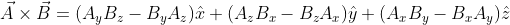(10)

The Dot Product
(a) When B < A.
(b) When B > A.
Figure 3: The projection of vectoron to vector.

Having discussed the perpendicularity of two vectors, it's natural to ask if there's a similar measure of the parallelity of two vectors. There are two ways of doing this. The way I'll do it first is explicitly geometrical, the second way is only implicitly geometrical.

Say we have two vectorsandagain, and we want to know how much ofis pointing (projected) along. From Fig. 3 we see that this is equal to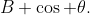(11)
Similarly, the amount ofthat is projected alongis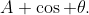(12)
Now, it would be nice if we could have one statement which somehow combined the these two statements and gave a measure both of how much ofis pointing alongand of how much ofis pointing along; that is, a measure of how much these two vectors point in the same direction. Additionally, since (2) used a multiplicative combination of the two vectors as a measure of perpendicularity, we'll try a similar multiplicative measure here, as well.

If we multiply (11) byand (12) bywe can write a single, symmetric statement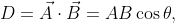(13)
and say it's the result of a ``dot product'' ofand, which amounts to multiplying together the parallel parts of two vectors. Here, too, if we don't know the angle between them, we're not out of luck. For a vector written in component form, it's straightforward to multiply the parallel parts together: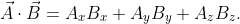(14)

However, unlike the cross product which gave us an actual area with a natural direction, this area-like structure is actually a measure of ``non-area'' and doesn't really have a natural direction. Although we could, completely arbitrarily, define a direction for this dot product,4 and thus make it a vector as well, to the best of my knowledge such a quantity does not have any uses in physics, so we'll leave it alone and treat it only as a number (scalar).

Alternatively, we know that the largest area possible between two vectors occurs when they are perpendicular to each other, where the area is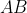(you can also see this from (3)). If we are interested in the maximal ``amount perpendicular'' we can write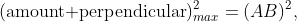(15)
where they are squared to take care of sign problems. Now, when they are completely parallel there is no area, and we're left only with non-area, which, also, can't be larger than the total maximum area, so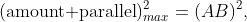(16)
as well.

Then using a rough analogue to the Pythagorean theorem we see that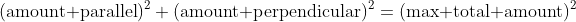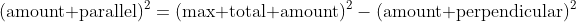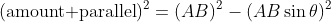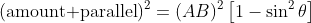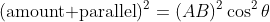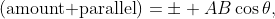(17)
which, choosing the positive root, is the same as (13).

References
 F.W. Sears and M.Z. Zemansky. University Physics. Addison-Wesley, Reading, MA, 2nd edition, 1955.
 D. Halliday amd R. Resnick and J. Walker. Fundamentals of Physics. John Wiley & Sons, Inc., 7 edition, 2005.
 G.R. Fowles and G.L. Cassiday. Analytical Mechanics. Thomson Brooks/Cole, Belmont, CA, 7th edition, 2005.
 J.R. Reitz, F.J. Milford, and R.W. Christy. Foundations of Electromagnetic Theory. Addison-Wesley, 4th edition, 1992.
 D.J. Griffths. Introduction to Quantum Mechanics. Pearson Prentice Hall, 2nd edition, 2005.
 J. Stewart. Multivariable Calculus. Brooks/Cole Publishing Company, Pacific Grove, CA, 4th edition, 1999.
 W. Lewin. Lec 3 | 8.01 Physics I: Classical Mechanics, Fall 1999 [online]. Available from:http://www.youtube.com/watch?v=fwNQKjTj-0w#t=13m45s [cited 16 March 2009].
 C.T.J. Dodson and T. Poston. Tensor Geometry: The Geometric Viewpoint and its Uses. Springer, 2nd edition, 1997.

1 Of course, not all first semester physics students even know what a determinant is, but that is not my point.
2 Work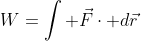, and torque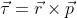3Another way to approach this is to start by calculating the area, and then explain that this can also be viewed as a measure of perpendicularity.
4 i.e. along eitheror, or along a line midway between them, or perpendicular to them, or some other arbitrary choice